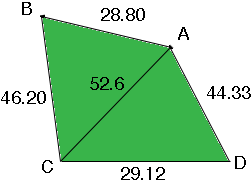SEARCH HOMEMath Central Quandaries & QueriesQuestion from Greg: Hi my name is Greg. I have a piece of property I am trying to buy, but need to find out the square feet of it first. The four sides are unequal lengths and none at a right angle or parallel. Here are the measurement in feet. The base of the square is 29.12 feet in width. The right side of the square is 44.33 feet high. The top of the square is 28.80 feet in width. The left side of the square is 46.20 feet high. I have not done this math in ages and really need your help. Best, GregHi Greg,

Unfortunately, knowing only the lengths of the sides is not enough information to find the area. I need to know something about the shape, either an angle or the length of a diagonal. There are many different shaped properties with different areas that have exactly these side lengths. There is more information on this at mathcentral.uregina.ca/QQ/database/QQ.09.03/dave1.html

Harley

Greg wrote back.

Harley,

The diagonal length is 52.6 feet.

Thanks,

Greg

Greg,

I drew a diagram of your property and labeled the corners. Unfortunately I didn't ask you to tell me which diagonal you measured so, for the moment, I chose one.Dimensions in feet

The property is divided into two triangles and I can find the area of these triangles using Heron's Formula. I used Heron's formula and found

area of triangle ABC = 663.5 sq ft
area of triangle ACD = 645.3 sq ft
area of the property = 1308.79 sq ft

If the 52.6 ft diagonal joins B and D the I get

area of triangle ABD = 638.3 sq ft
area of triangle BCD = 670.5 sq ft
area of the property = 1308.82 sq ft

It is surprising they are so close,
HarleyMath Central is supported by the University of Regina and The Pacific Institute for the Mathematical Sciences.